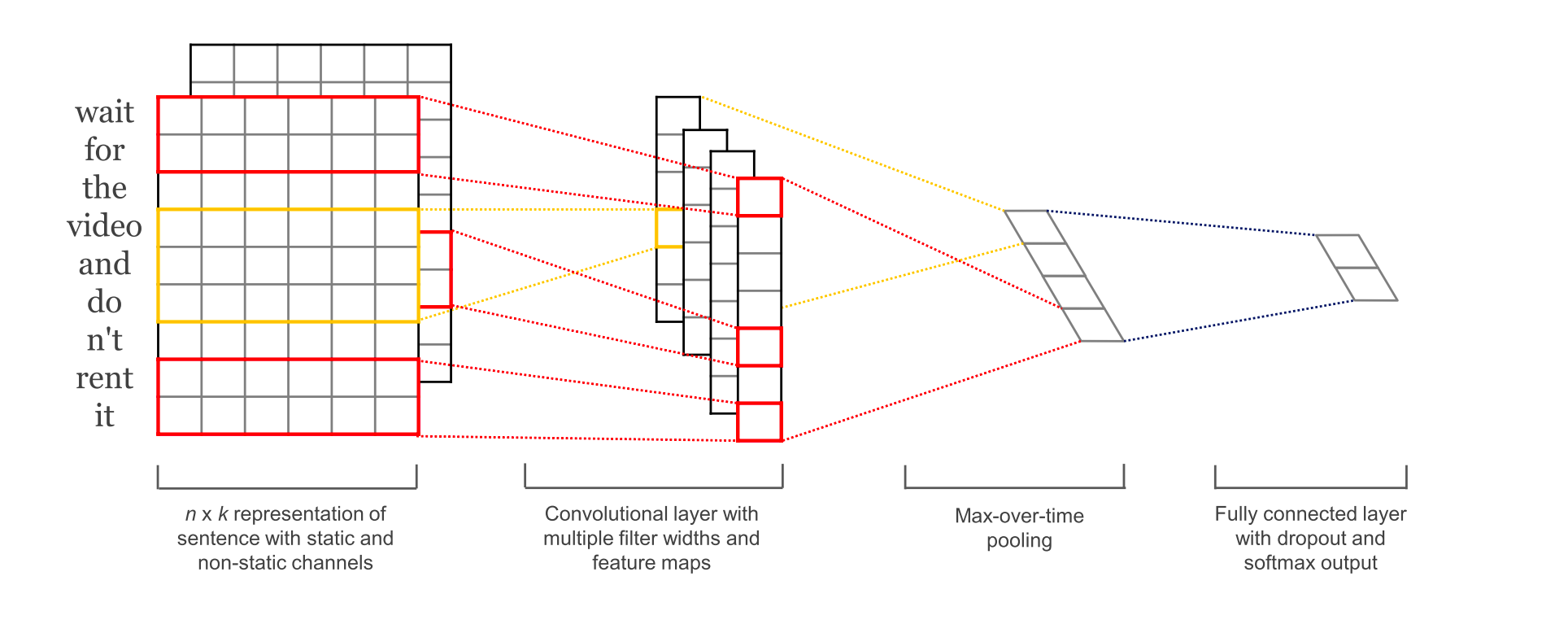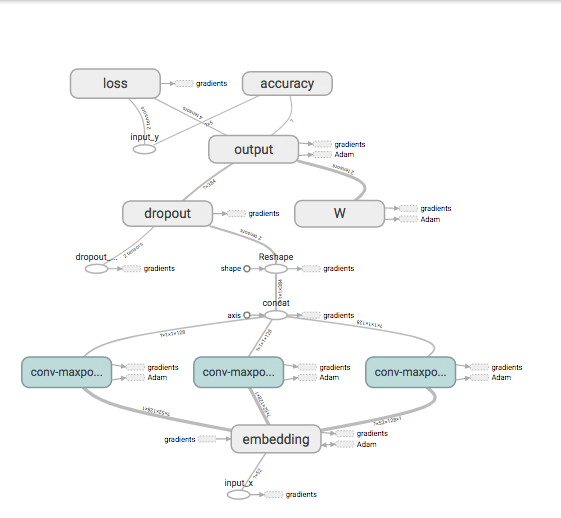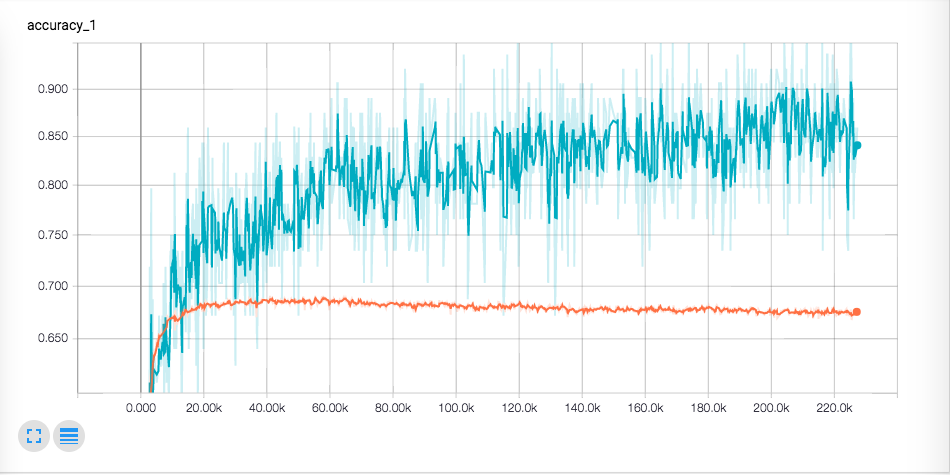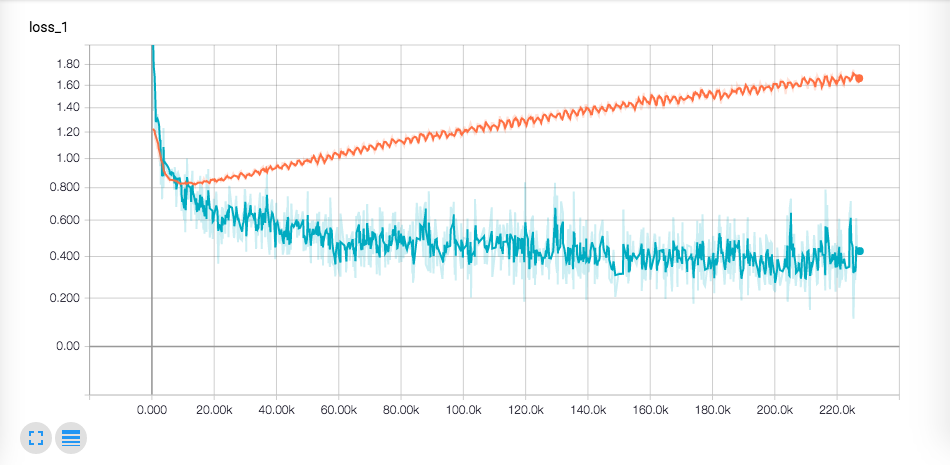# 使用 Tensorflow 构建 CNN 进行情感分析实践 - 腾讯云社区 - 腾讯云

## 1 导论

Web挖掘中的情感分析类问题，其实是一个分类问题。而CNN可以用来处理分类任务，就是在最终的softmax函数计算属于各个类的概率，并归属到概率最大的类。

## 2 系统

### 2.2 网络### 2.3 代码实现

``````classTextCNN(object):def__init__(
self, sequence_length, num_classes, vocab_size,
embedding_size, filter_sizes, num_filters, l2_reg_lambda=0.0):``````

``````# Embedding layerwith tf.device('/cpu:0'), tf.name_scope("embedding"):self.W = tf.Variable(
tf.random_uniform([vocab_size, embedding_size],-1.0,1.0),
name="W")self.embedded_chars = tf.nn.embedding_lookup(self.W,self.input_x)self.embedded_chars_expanded = tf.expand_dims(self.embedded_chars,-1)``````

``````pooled_outputs = []
for i, filter_size in enumerate(filter_sizes):
with tf.name_scope("conv-maxpool-%s" % filter_size):# Convolution Layerfilter_shape = [filter_size, embedding_size, 1, num_filters]
W = tf.Variable(tf.truncated_normal(filter_shape, stddev=0.1), name="W")
b = tf.Variable(tf.constant(0.1, shape=[num_filters]), name="b")
conv = tf.nn.conv2d(
self.embedded_chars_expanded,
W,
strides=[1, 1, 1, 1],
name="conv")# Apply nonlinearityh = tf.nn.relu(tf.nn.bias_add(conv, b), name="relu")# Maxpooling over the outputspooled = tf.nn.max_pool(
h,
ksize=[1, sequence_length - filter_size + 1, 1, 1],
strides=[1, 1, 1, 1],
name="pool")
pooled_outputs.append(pooled)``````## 3 实验结果`tensorboard --logdir ./runs/1497715905/summaries/`## 使用 Tensorflow 构建 CNN 进行情感分析实践 - 腾讯云社区 - 腾讯云

- -
Web挖掘中的情感分析类问题，其实是一个分类问题. 而CNN可以用来处理分类任务，就是在最终的softmax函数计算属于各个类的概率，并归属到概率最大的类. 本次实验参照的是Kim Yoon的论文Convolutional Neural Networks for Sentence Classification.

- - Soul Joy Hub

- - IT瘾-dev

- - IT瘾-tuicool

- - V2EX

## Zite 正和 CNN 谈判收购事宜？

- Leo - 爱范儿 · Beats of Bits

- - IT瘾-dev